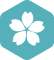### 什么是线性方程，什么是非线性方程啊？djhdu

Matlab利用牛顿迭代法求解非线性方程

Matlab 解非线性方程

Matlab 算是一个比较好的计算工具了，偶尔可以用用，现解方程组如下： syms a b c f1 = a*exp(0)-c f2 = a*exp(481*b)-c-2107/16 f3 = a*exp(962*b)-c-2107 [a b c] = solve([f1 f2 f3]) 之后输出： a = 2107/...

MATLAB线性方程组求解

1. 非线性方程的求解 1.1 简介 　非线性方程是指含有指数和余弦函数等非线性函数的方程，例如，ex−cos(πx)=0ex−cos(πx)=0e^x-cos(\pi x)=0 与线性方程相比，无论是解的存在性，还是求解的计算公式，非线性...

matlab-求解非线性方程/方程组

python牛顿法解非线性方程组_牛顿迭代法解非线性方程组（MATLAB版）

C语言牛顿迭代法求解非线性方程

Octave:5.1.0 参考书籍：OctaveAtBFH.pdf（自行网上搜索） 需要两个文件： 函数文件fsolveNewton.m，函数名和文件名一定要对应 脚本文件nonlinear_test.m 参考书籍OctaveAtBFH第72页内容： fsolveNewton.m 程序 ...

matlab-fsolve解非线性方程组一例

MATLAB牛顿法求解非线性方程组1

function Newton() x0=[0.1;0.5]; x1=x0-inv(myJacobi(x0))*myfun(x0); while norm(x1-x0)>1e-3 x0=x1; x1=x0-inv(myJacobi(x0))*myfun(x0); end x1 end function f=myfun(x) ...f1=(15*x1+10...

matlab-fsolve求解非线性方程/带参数

matlab fsolve解非线性方程组_MATLAB求解方程

MATLAB求解方程(1)roots函数功能：用于求解多项式方程的根，以列矢量的形式返回多项式的根。调用格式：r=roots(p)，p是多项式系数按降幂排列所形成的向量。示例：求方程3x2-2x-4=0的根。代码：p=[3,-2,-4];r=roots(p...

【Matlab学习手记】非线性方程组求解：牛顿法

1.前言 算法学习这部分，自从离开了数论后，程序效率可提升的地方变少了，因此看起来，好像没意思了……换句话说，之前那种通过尝试不同语句、不同求解思路来极大提升程序效率的快感没了……这好像也间接导致了我...

【Matlab学习手记】非线性方程求解：二分法

Python第三方库scipy.optimize中的fsolve和root这两个函数可用于非线性方程组的求解。但其更适用于结构形式稍显简单的方程组求解。对于多变量结构复杂的非线性方程组的求解操作起来稍显困难。因此，自己动手编写求解...

python求解各种复杂的线性/非线性方程